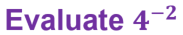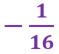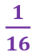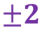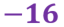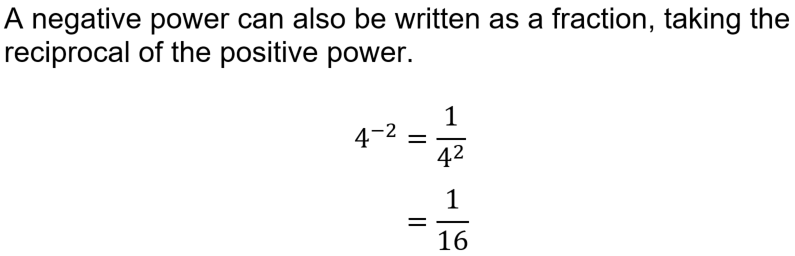What do you know about indices and roots? Find out in this quiz.

# Indices and Roots (F)

Using INDICES, or powers, is a shorthand way of showing that a number (or a variable) has been multiplied by itself. Taking the root of a number is this process in reverse. Take this GCSE Maths quiz to see how well you can cope with all the little rules! You will know that the I in BIDMAS stands for Indices (or perhaps you’re more familiar with BODMAS, where the I is replaced with an O for Orders). Have a read of our BIDMAS Order of Operations page for a full recap of this subject.

You should know about the idea of multiplying a number by itself, or squaring it. The reverse process, taking the square root, involves finding a number that, when multiplied by itself, gives you the number you started with. Mathematicians developed the notation to use a small, raised power as a shorthand way of writing out repeated multiplication. This has been developed to include negative and fractional indices, and you should be familiar with all of them.

Learn the laws of indices to help you perform calculations involving roots and powers, and remember that ‘anything to the power of zero is always equal to 1’.

Standard form, also known as scientific notation, is a useful way of writing very large or very small numbers. Part of a number written in standard form includes 10 to the power of something. This works because big numbers, like 1,000,000 (1 million), can be written as 106. We might not be able to visualise really big numbers, but standard form at least allows us to perform calculations without getting caught up in a pile of zeroes!

Question 1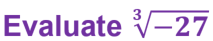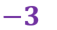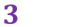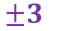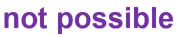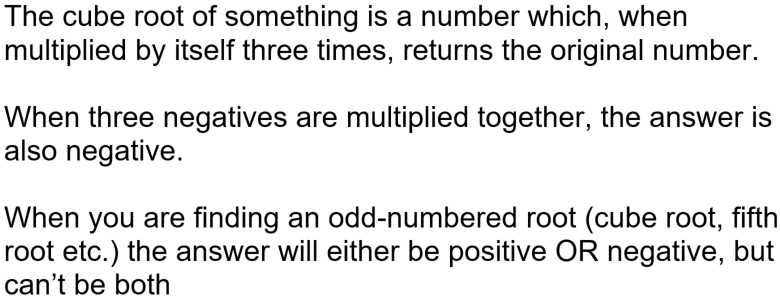Question 2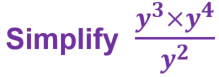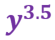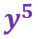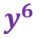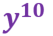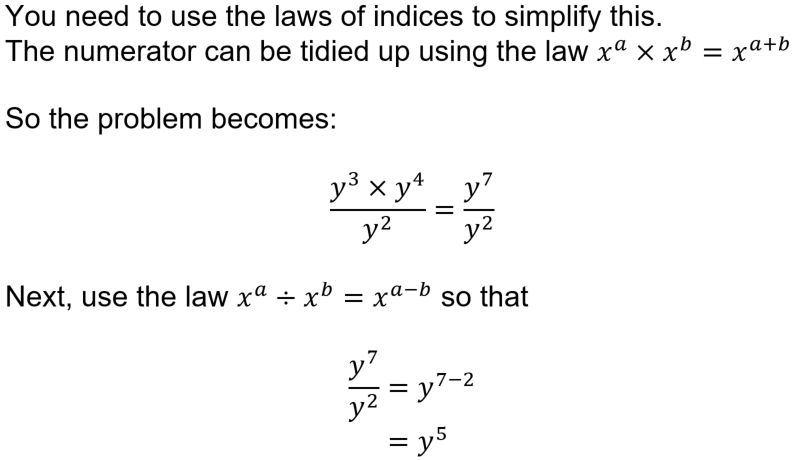Question 3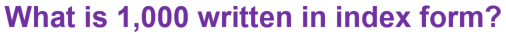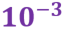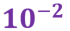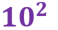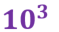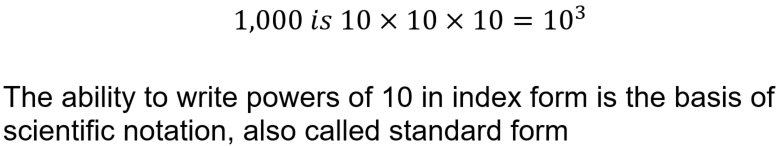Question 4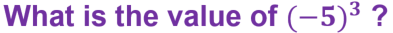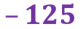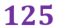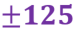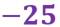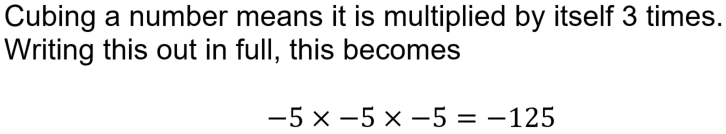Question 5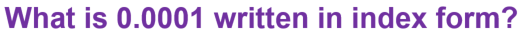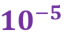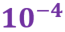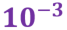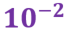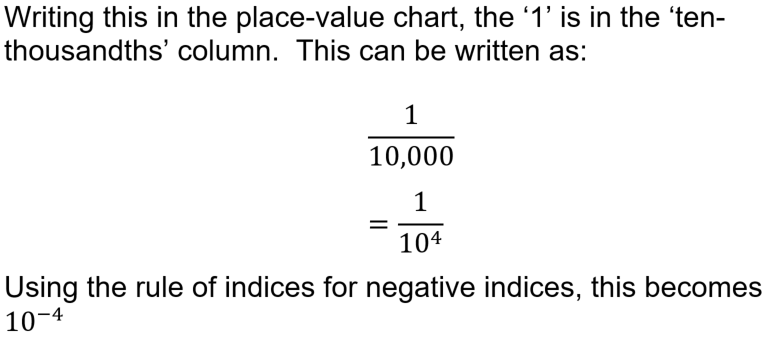Question 6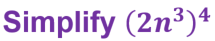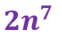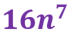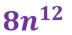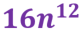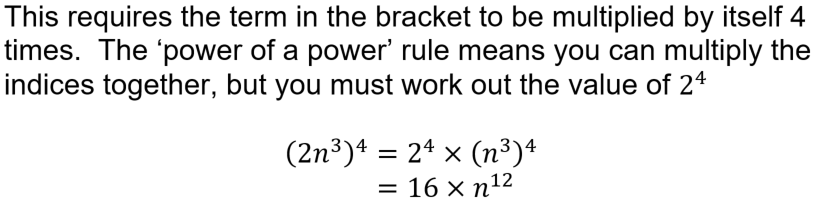Question 7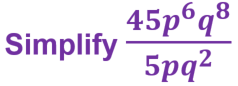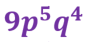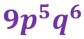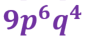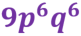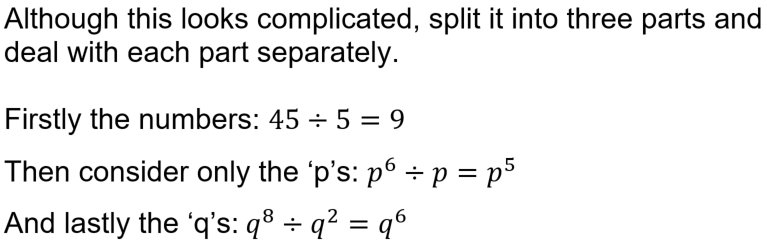Question 8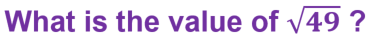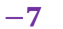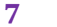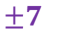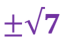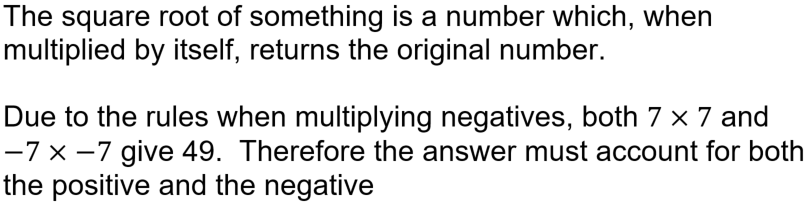Question 9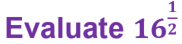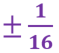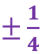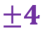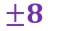Question 10